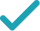Type a math problemSolve for xSolution Steps
Divide both sides by . Since is , the inequality direction remains the same.
Divide by to get .
Calculate the square root of and get . Rewrite as .
Inequality holds for .
Rewrite as .
GraphGraph Inequality
Graph Both Sides in 2D
x^{2}\geq \frac{50}{2}
Divide both sides by 2. Since 2 is >0, the inequality direction remains the same.
x^{2}\geq 25
Divide 50 by 2 to get 25.
x^{2}\geq 5^{2}
Calculate the square root of 25 and get 5. Rewrite 25 as 5^{2}\approx 25.
|x|\geq 5
Inequality holds for |x|\geq 5.
x\leq -5\text{; }x\geq 5
Rewrite |x|\geq 5 as x\leq -5\text{; }x\geq 5.